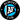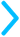## Mixed Numbers & Fractions

A mixed number or mixed fraction is a whole number plus a fraction, like this:

$2\frac{3}{4}$

It's equivalent to:

$2\frac{3}{4} = 2 + \frac{3}{4}$

But, apparently, someone came in social-network style and decided to drop the plus sign to make things cleaner.The key to mixed numbers and fractions is to remember that hidden plus sign. Try out our convertors below to see how to convert between a fraction and a mixed number.

### Mixed Number to Fraction Calculator

Enter a mixed number and walk through the steps of converting it to an improper fraction.

### Fraction to Mixed Number Calculator

Enter an improper fraction and walk through the steps of converting it to a mixed number.